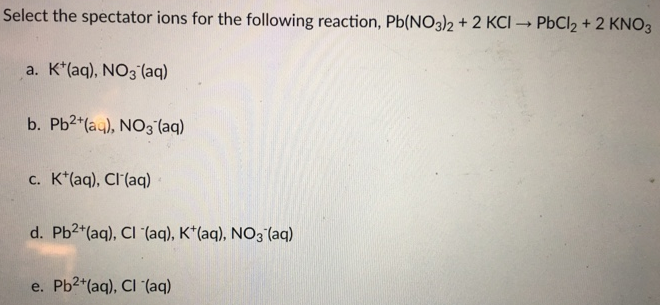# Problem: Select the spectator ions for the following reaction, Pb(NO3)2 + 2 KCI → PbCI2 + 2KNO3 a. K+(aq), NO3- (aq) b. Pb2+(aq), CI- (aq) c. K+(aq), CI- (aq) d. Pb2+(aq), CI- (aq), K+ (aq), NO3- (aq) e. Pb2+(aq).CI- (aq)

###### FREE Expert Solution

The net ionic and total ionic equation for this reaction are:

87% (299 ratings)###### Problem Details

Select the spectator ions for the following reaction, Pb(NO3)2 + 2 KCI → PbCI2 + 2KNO3

a. K+(aq), NO3- (aq)

b. Pb2+(aq), CI- (aq)

c. K+(aq), CI- (aq)

d. Pb2+(aq), CI- (aq), K+ (aq), NO3- (aq)

e. Pb2+(aq).CI- (aq)Frequently Asked Questions

What scientific concept do you need to know in order to solve this problem?

Our tutors have indicated that to solve this problem you will need to apply the Complete Ionic Equations concept. You can view video lessons to learn Complete Ionic Equations. Or if you need more Complete Ionic Equations practice, you can also practice Complete Ionic Equations practice problems.

What professor is this problem relevant for?

Based on our data, we think this problem is relevant for Professor Yates' class at UK.